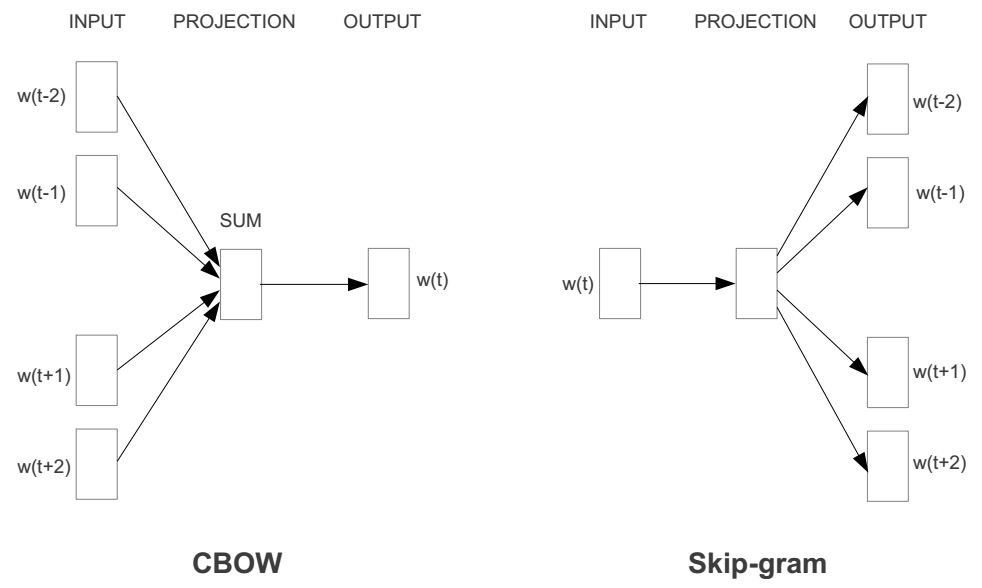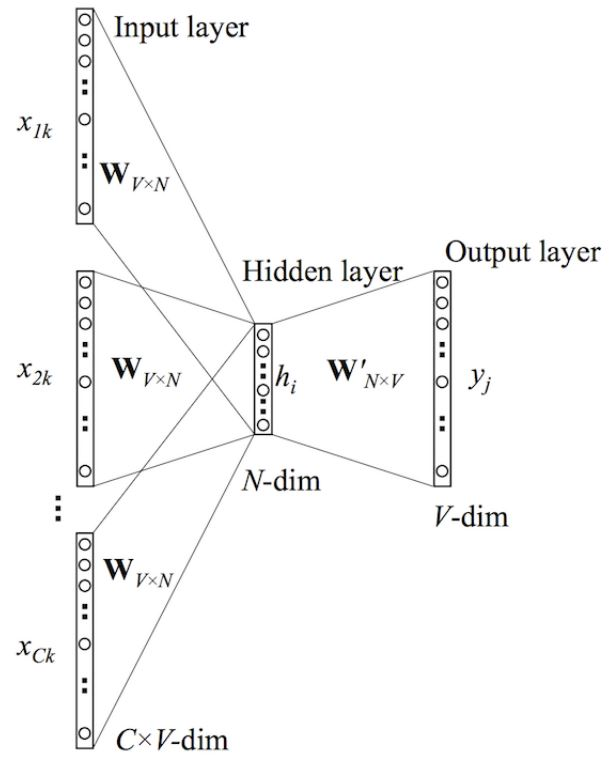# cbow模型详解_老C模型

cbow模型详解_老C模型引言前面我分析了Word2vec的一种模型叫做skip-gram模型。在这篇文章中，我将讲述另一个word2vec模型——连续词袋模型（CBOW）模型。如果你理解skip-gram模型，那么接下来的CBOW模型就更好理解了，因为两者模型互为镜像。我们先来看看CBOW模型与skip-gram模型对比图：如何，这是不是镜像关系？所以接下来的讲解也会和skip-gram那篇文章极其类似。前向传播接

## 引言## 前向传播x 1 x_1 ,…, x C x_C }组成，其中窗口大小为C，词汇表大小为V。隐藏层是N维的向量。最后输出层是也被one-hot编码的输出单词 y y 。被one-hot编码的输入向量通过一个 V × N V\times N 维的权重矩阵 W W 连接到隐藏层；隐藏层通过一个 N × V N\times V 的权重矩阵 W ′ W^{‘} 连接到输出层。

• 第一步就是去计算隐藏层 h h 的输出。如下：
h = 1 C W ⋅ ( ∑ i = 1 C x i ) ( 1 ) h = \frac{1}{C}W\cdot (\sum_{i=1}^C x_i)\tag{$1$}
该输出就是输入向量的加权平均。这里的隐藏层与skip-gram的隐藏层明显不同。
• 第二部就是计算在输出层每个结点的输入。如下：
u j = v w j ′ T ⋅ h ( 2 ) u_{j}=v^{‘T}_{wj}\cdot h\tag{$2$}
其中 v w j ′ T v^{‘T}_{wj} 是输出矩阵 W ′ W^{‘} 的第 j j 列。
• 最后我们计算输出层的输出，输出 y j y_j 如下：
y c , j = p ( w y , j ∣ w 1 , . . . , w c ) = e x p ( u j ) ∑ j ′ = 1 V e x p ( u ′ j ) ( 3 ) y_{c,j} =p(w_{y,j}|w_1,…,w_c) = \frac{exp(u_{j})}{\sum^V_{j^{‘}=1}exp(u^{‘}j)}\tag{$3$}

## 通过BP（反向传播）算法及随机梯度下降来学习权重

• 首先就是定义损失函数，这个损失函数就是给定输入上下文的输出单词的条件概率，一般都是取对数，如下所示：
E = − l o g p ( w O ∣ w I ) ( 4 ) E = -logp(w_O|w_I)\tag{$4$}
= − v w o T ⋅ h − l o g ∑ j ′ = 1 V e x p ( v w T j ′ ⋅ h ) ( 5 ) = -v_{wo}^T\cdot h-log\sum_{j^{‘}=1}^Vexp(v^T_w{_{j^{‘}}}\cdot h)\tag{$5$}
接下来就是对上面的概率求导，具体推导过程可以去看BP算法，我们得到输出权重矩阵 W ′ W^{‘} 的更新规则：
w ′ ( n e w ) = w i j ′ ( o l d ) − η ⋅ ( y j − t j ) ⋅ h i ( 6 ) w^{‘(new)} = w_{ij}^{‘(old)}-\eta\cdot(y_{j}-t_{j})\cdot h_i\tag{$6$}
同理权重 W W 的更新规则如下：
w ( n e w ) = w i j ( o l d ) − η ⋅ 1 C ⋅ E H ( 7 ) w^{(new)} = w_{ij}^{(old)}-\eta\cdot\frac{1}{C}\cdot EH\tag{$7$}

## 参考文献

 Mikolov T, Chen K, Corrado G, et al. Efficient Estimation of Word Representations in Vector Space[J]. Computer Science, 2013.（这篇文章就讲了两个模型：CBOW 和 Skip-gram）
 Mikolov T, Sutskever I, Chen K, et al. Distributed Representations of Words and Phrases and their Compositionality[J]. 2013, 26:3111-3119.（这篇文章针对Skip-gram模型计算复杂度高的问题提出了一些该进）
 Presentation on Word2Vec（这是NIPS 2013workshop上Mikolov的PPT报告）

【正版授权，激活自己账号】： Jetbrains全家桶Ide使用，1年售后保障，每天仅需1毛

【官方授权 正版激活】： 官方授权 正版激活 支持Jetbrains家族下所有IDE 使用个人JB账号...

(0)### 相关推荐

• #### Linux下重启tomcat

linux重启tomcat命令1，进入到tomcat的bin目录下使用ls命令，查看自己的目录文件cd/opt/tomcat_test/bin/（cd后面接自己的环境目录）2、启动tomcat运行bin目录下的启动命令脚本shstartup.sh或者./startup.sh这样tomcat就启用了3.查看tomcat进程是否启动使用psaux|greptomca…

• #### 快速搭建静态网站生成器「建议收藏」

快速搭建静态网站生成器「建议收藏」快速搭建静态网站生成器下面有许多静态页面生成器，大家可以根据需求学以致用。快速搭建静态网站：https://www.staticgen.com/

• #### 解决ModuleNotFoundError: No module named ‘pip‘问题

解决ModuleNotFoundError: No module named ‘pip‘问题Python学习遇到小问题：ModuleNotFoundError:Nomodulenamed‘pip’今天想要装一下wxPython第三方库来写一下Python的GUI的时候发现cmd窗口下无法执行pip命令，想了想昨晚好像是pip命令行提示了我有新版本可以更新使用，更新之后也不成功，但昨晚没有怎么理会，以为没事，但今早起来一看发现pip命令都用不了了，出现了ModuleNotFoun…

• #### PHP怎么获取系统信息和服务器详细信息

PHP怎么获取系统信息和服务器详细信息

• #### toArray方法的使用简记「建议收藏」